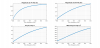Reports 1-1 of 1
vardaro - 18:19 Monday 24 January 2022 (54590) Print this report
Extraction of a signal for DARM High frequency pole frequency

In the tuning of the ITF working point in CARM null we found out that moving the SR_MAR_TX set point affects the position of the DARM pole at high frequency  (see elog 54460) . The adjustment of the ITF set point is done injecting noise in DARM. Thus we are studing how to extract information on the DARM high frequency pole frequency using two lines injected in DARM.

The first line is the same line used to measure the DARM UGF at 74.4 Hz. An additional line was added at 304.4 Hz the amplitude of this line is 4e-6.

We cmputed the magnitude of each line in the DARM oltf and we did the ratio of them. We changed the frequency of the DARM high frequency pole from 100Hz to 400 Hz and we plot:

• the magnitude of the 74.4Hz line (up left plot)
• the magnitude of the 304.4Hz line (up right plot)
• the ratio between the magnitude of the two lines (bottom left plot)
• the square of the ratio between the magnitude of the two lines multuplied by a factor (bottom right plot)

The signal in the bottom right plot seems to scale linearly with the pole frequency thus is a good candidate as monitor channel for DARM high frequency pole. The channel was implemented in Acl Last week (elog 54516). The name of this signal is LSC_DARM_HF_pole_mag_sqrt (ratio between the two lines)    DARM_HF_pole_mag (square of the ratio between the two lines).

Images attached to this report×

×